## Thursday, 27 January 2022

SBI Clerk Day 3 Reasoning Online Test 3

1.  Directions(Q.1 to Q.5 ):

Study the following information carefully to answer these questions.

A group of people has six family members and an advocate. These are L, M, N, O, P, Q and R and having different professions. Each one of them is a journalist, businessman, architect, doctor and pilot but not necessarily in this order. There are three males and three females in the family out of which there are two married couples. M is a businessman and is the father of P. N is a housewife and is daughter-in-law of O. L is neither a pilot nor a journalist. R is an advocate. N is not the mother of P and O is not married to M. No lady is a journalist.

Who among the following family members is an architect ?

a
O
b
P
c
Can’t be determined
d
L
e
None of theseView Answer
Explanation :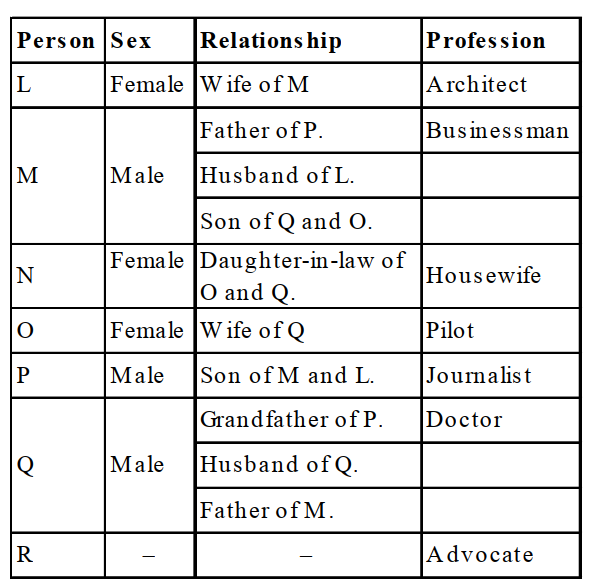Two married couples : LM and QO.

2.

How is Q related to O ?

a
Mother
b
Mother-in- law
c
Father
d
Son - in - law
e
None of theseView Answer
Explanation :Two married couples : LM and QO.

3.

Which of the following groups represents the three ladies in the group ?

a
N, P, L
b
P, L, N
c
L, N, O
d
O, P, L
e
None of theseView Answer
Explanation :Two married couples : LM and QO.

4.

Which of the following is the profession of P ?

a
Journalist
b
Architect
c
Pilot
d
Architect or pilot
e
None of theseView Answer
Explanation :Two married couples : LM and QO.

5.

Who is married to Q ?

a
L
b
Can’t be determined
c
O
d
N
e
None of theseView Answer
Explanation :Two married couples : LM and QO.

6.

In a certain code language ’Ka Bi Pu Ya’ means ’You are very intelligent’; ’Ya Lo Ka Wo’ means ’They seem very intelligent’; ’La Pu Le’ means ’You can see’ and ’Sun Pun Yun Ya’ means ’how intelligent she is’, In that language, which of the following words means ’are’?

a
Bi
b
Pu
c
Ka
d
Ya
e
None of theseView Answer
Explanation :

From first 2 sentences ’Ka Ya’ means ’very intelligent’.

From 1st and 3ʳᵈ sentences ’Pu’means ’you’

∴ In first sentence ’are’ means ’Bi’

7.

Rama remembers that she met her brother on Saturday, which was after the 20th day of a particular month. If the 1st day of that month was Tuesday, then on which date did Rama meet her brother ?

a
24th
b
25th
c
26th
d
23rd
e
None of theseView Answer
Explanation :

1st of month was Tuesday, hence the date on first Saturday was 5th.

Hence the other Saturdays of the month are 12, 19, 26. Rama met her brother on 26th.

8.

Ankit is related to Binny and Chinky, Daizy is Chinky’s mother. Also Daizy is Binny’s sister and Aruna is Binny’s sister. How is Chinky related to Aruna?

a
Cousin
b
Sister
c
Aunt
d
Niece
e
None of theseView Answer
Explanation :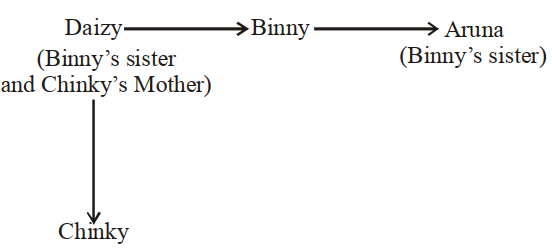9.

Which is the third number to the left of the number which is exactly in the middle of the following sequence of numbers?

1 2 3 4 5 6 7 8 9 2 4 6 8 9 7 5 3 9 8 7 6 4 3 2 1

a
2
b
3
c
5
d
6
e
None of theseView Answer
Explanation :

There are 25 numbers in the given sequence.

So, middle number = 13th number = 8.

Clearly, the third number to the left of this 8 is 2

10.

In a row of girls, Rita and Monika occupy the ninth place from the right end and tenth place from the left end, respectively. If they interchange their places, then Rita and Monika occupy seventeenth place from the right and eighteenth place form the left respectively. How many girls are there in the row?

a
25
b
27
c
d
26
e
None of theseView Answer
Explanation :

Total no. of girls = 17 + 10 – 1 or 18 + 9 – 1 = 26

11.

If it is possible to make only one such number with the first, the fourth and the sixth digits of the number 531697 which is the perfect square of a two digit even number, which of the following will be the second digit of the two digit even number. If no such number can be made, give ’@’ as the answer and if more than one such number can be made, give ’©’ as the answer.

a
6
b
@
c
2
d
4
eView Answer
Explanation :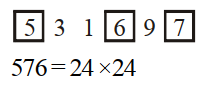12.

If ’K’ denotes ’×’, ’B’ denotes ’÷’, ’T’ denotes ’–’ and ’M’ denotes ’+’, then –

40 B 8 T 6 M 3 K 4 = ?

a
19
b
11
c
23
d
–31
e
None of theseView Answer
Explanation :

40 B 8 T 6 M 3 K 4 = ?

⇒ ? = 40 + 8 – 6 + 3 × 4

⇒ ? = 5 + 6 – 12 = 11

13.

In a certain code IDEAS is written as HEDBR and WOULD is written as VPTMC. How will RIGHT be written in the same code ?

a
SHHGU
b
QJHIS
c
QJFIU
d
QJFGS
e
QJFISView Answer
Explanation :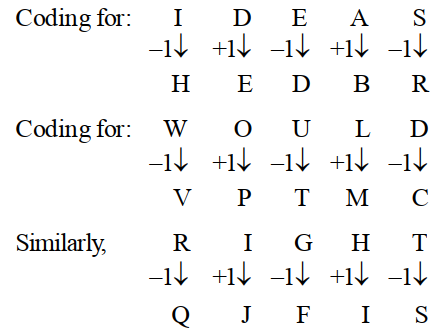14.

In a certain code JOURNEY is written as TNISZFO. How is MEDICAL written in that code?

a
EFNJMBD
b
CDWDBM
c
LDCJMBD
d
CDLJMBD
e
None of theseView Answer
Explanation :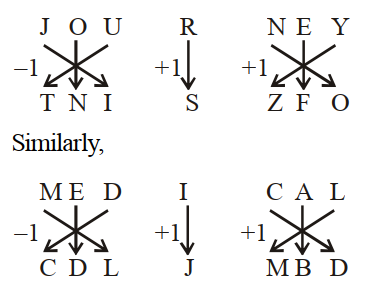15.

If the alphabet is written in the reverse order and every alternate letter starting with Y is dropped, which letter will be exactly in the middle of the remaining letters of the alphabet.

a
M or O
b
N
c
O
d
M
e
None of theseView Answer
Explanation :

Cancelling every second letter after reversing the

alphabet the series becomes.

Z X V T R P N L J H F D B

The middle letter is N

16.  Directions(Q.16 to Q.20 ):

Use the following series of elements (alpha-number-symbol) to answer these questions. Every two-digit number (given in brackets) is to be treated as single number.

2 ★ 8 5 6 B 9 $Q 3 E 1 7 R D 4 £ (13) U ⬤ K (18) A (14) P Four of the following five groups of elements are alike in a certain way and so form a group. Which is the one the does not belong to that group ? a 2 ★ 8 b 1 3 E c 56 B d Q$ 9
e
D R 7View Answer
Explanation :

Other groups consist of consecutive elements.

17.

If each of the letters in the above series of elements is given a value equivalent to its serial number in the English alphabet, what will be the difference between the sum of the consonants and the sum of the vowels used in the series ?

a
109
b
82
c
27
d
41
e
None of theseView Answer
Explanation :

The letters used in the series are B Q E R D U K A P.

Sum of the positions of consonants according to

alphabet = 2 + 17 +18 + 4 + 11 + 16 = 68. Similarly, sum

of the vowels’ positions = 5 + 21 + 1 = 27. Hence required

difference = 68 – 27 = 41

18.

If each alphabet has a value of zero, each symbol (i.e.,★ , $and ⬤ ) has a value equivalent to the square of the numeral that immediately precedes the symbol or the value of 1 if it is not immediately preceded by a numeral, what will be the sum of the values of the first 10 elements of the series starting from the left end ? a 46 b 107 c 118 d 79 e None of theseView Answer Answer & Explanation Answer : Option C Explanation : 2 + 22 + 8 + 5 + 6 + 0 + 9 + 92 + 0 + 3 = 118 Where value of alphabet = 0; symbol = square of the previous number; number = the value itself. 19. Which of the following groups of elements will come in the place of the question-mark in the series of elements given below? 6 8 2$ B 5 E Q 9 ? £ D 7

a
⬤ (13) 4
b
⬤ (13) £
c
4 (13) ⬤
d
U £ D
e
None of theseView Answer
Explanation :

First element of each group follows the order + 3, + 3, +3... and so on.

Middle element and last element also follow the same trend. Hence (?) should be replaced by R 13.

20.

BQ in the above series is related in ER in a similar way as AP is related to

a
U ⬤
b
RD
c
KA
d
Q 3
e
6 BView Answer
Explanation :

Number of elements between B and Q is the same as that between E and R. Similarly, number of elements between A and P is the same as that between K and A.

21.  Directions(Q.21 to Q.25 ):

In each question below are three statements followed by three conclusions numbered I, II and III. You have to take the three given statements to be true even if they seem to be at variance from commonly known facts and then decide which of the answers (a), (b), (c), (d) and (e) is the correct answer and indicate it on the answer sheet.

Statements:

Some spectacles are lenses.

Some lenses are frames.

All frames are metals.

Conclusions:

I. Some lenses are metals

II. Some metals are spectacles.

III. Some frames are spectacles

a
Only III follows
b
Only I and either II or III follow
c
Only I follows
d
Only I and II follow
e
None of theseView Answer
Explanation :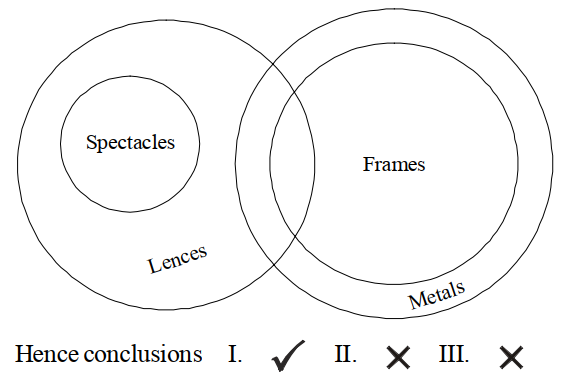22.

Statements:

All stones are rocks.

Some rocks are bricks.

Some bricks are cement.

Conclusions:

I. Some cements are rocks.

II. Some bricks are stone

III. Some stones are cement

a
Only I and either II or III follow
b
Only I and II follow
c
All follow
d
Only either II or III follows
e
None of theseView Answer
Explanation :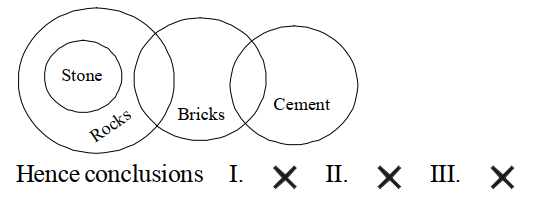23.

Statements:

All trees are flowers.

Some flowers are leaves.

No leaf is bud

Conclusions:

I. No bud is a flower.

II. Some buds are flowers.

III. Some leaves are trees.

a
Only II and III follow
b
Either I or II and III follow
c
Only either I or II follows
d
Only III follows
e
None of theseView Answer
Explanation :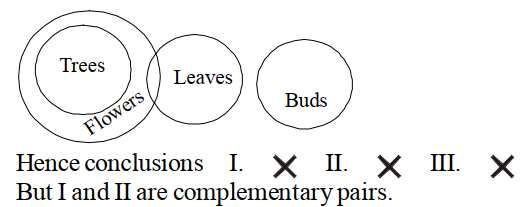24.

Statements:

Some chairs are tables.

Some tables are drawers.

all drawers are shelf.

Conclusions:

I. Some shelves are tables.

II. Some drawers are chairs.

III. Some shelves are drawers.

a
All I, II and III follow
b
Only I and either II or III follow
c
Only II and either I or III follow
d
Only I and III follow
e
None of theseView Answer
Explanation :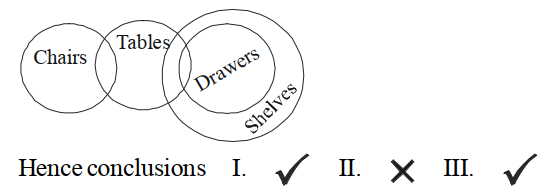25.

Statements:

All flats are buildings.

All buildings are bungalows.

All bungalows are apartments.

Conclusions:

I. Some apartments are flats.

II. All flats are bungalows.

III. Some bungalows are flats.

a
Only II and III follow
b
None follows
c
Only I and II follow
d
Only I and III follow
e
All I, II and III followView Answer
Explanation :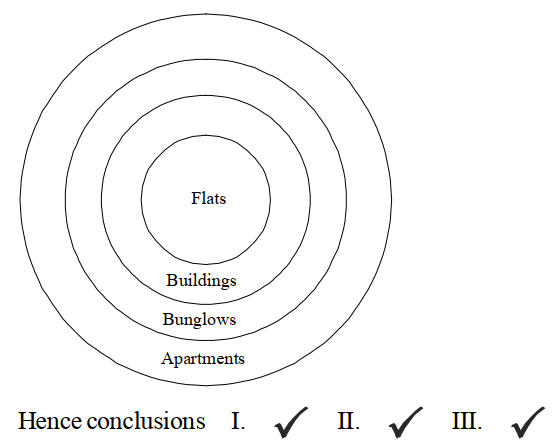26.  Directions(Q.26 to Q.30 ):

Read the following information carefully and answer the questions that follow: At a party, A, B, C, D and E are sitting in a circle. The group comprises a professor, an industrialist and a businessman. The businessman is sitting in between the industrialist and his wife D. A, the professor is married to E, who is the sister of B. The industrialist is seated to the right of C. Both the ladies are unemployed.

What is A to B ?

a
Uncle
b
Can’t be determined
c
Brother
d
Brother-in-law
e
None of theseView Answer
Explanation :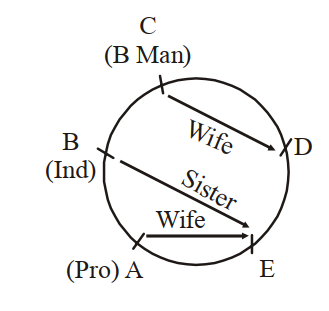A, the professor is married to E and E is the sister of B.

The wife of the industrialist is D. The industrialist is seated to the right of C. Thus, A and C cannot be industrialists.

Therefore, B is the industrialist and C is the business man.

Now, we come to the following deductions :

A – Professor B – Industrialist

C – Businessman D – Female, hence unemployed

E – Female, hence unemployed

A is the husband of E and E is the sister of B. Hence,

A is the brother-in-law of B.

27.

A is sitting to the right of

a
his wife
b
D
c
the industrialist
d
Can’t be determined
e
None of theseView Answer
Explanation :A, the professor is married to E and E is the sister of B.

The wife of the industrialist is D. The industrialist is seated to the right of C. Thus, A and C cannot be industrialists.

Therefore, B is the industrialist and C is the business man.

Now, we come to the following deductions :

A – Professor B – Industrialist

C – Businessman D – Female, hence unemployed

E – Female, hence unemployed

It cannot be determined, as no information has been provided in the paragraph about the sitting position of A.

28.

Who is the industrialist ?

a
A
b
B
c
Can’t be determined
d
D
e
None of theseView Answer
Explanation :A, the professor is married to E and E is the sister of B.

The wife of the industrialist is D. The industrialist is seated to the right of C. Thus, A and C cannot be industrialists.

Therefore, B is the industrialist and C is the business man.

Now, we come to the following deductions :

A – Professor B – Industrialist

C – Businessman D – Female, hence unemployed

E – Female, hence unemployed

As deduced earlier, B is the industrialist.

29.

Who in the group is unmarried?

a
Professor
b
Can’t be determined
c
d
Industrialist
e
None of theseView Answer
Explanation :A, the professor is married to E and E is the sister of B.

The wife of the industrialist is D. The industrialist is seated to the right of C. Thus, A and C cannot be industrialists.

Therefore, B is the industrialist and C is the business man.

Now, we come to the following deductions :

A – Professor B – Industrialist

C – Businessman D – Female, hence unemployed

E – Female, hence unemployed

It cannot be determined whether the businessman is married or unmarried

30.

Who among them must be graduate ?

a
C
b
E
c
B
d
A
e
None of theseView Answer